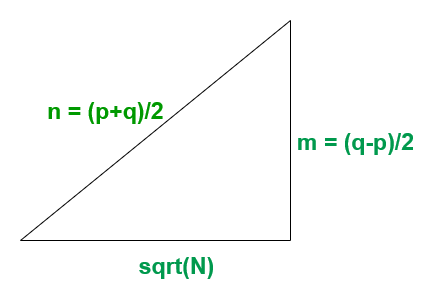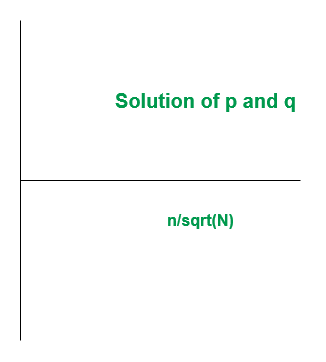# Check if a number is Prime, Semi-Prime or Composite for very large numbers

• Difficulty Level : Hard
• Last Updated : 18 Oct, 2021

Given a very large number N (> 150), the task is to check whether this number is Prime, Semi-Prime or Composite.
Example:

Input: N = 90000000
Output: Not Prime
Explanation:
we have (N-1)%6 = 89999999%6 = 1 and
(N+1)%6 = 90000001%6 = 5
Since n-1 and n+1 is not divisible by 6
Therefore N = 90000000 is Not Prime
Input: N = 7894561
Output: Semi-Prime
Explanation:
Here N = 7894561 = 71*111191
Since 71 & 111191 are prime, therefore 7894561 is Semi Prime

Attention reader! Don’t stop learning now. Get hold of all the important DSA concepts with the DSA Self Paced Course at a student-friendly price and become industry ready.  To complete your preparation from learning a language to DS Algo and many more,  please refer Complete Interview Preparation Course.

In case you wish to attend live classes with experts, please refer DSA Live Classes for Working Professionals and Competitive Programming Live for Students.

Approach:

• It can be observed that if n is a Prime Number then n+1 or n-1 will be divisible by 6
• If a number n exists such that neither n+1 nor n-1 is divisible by 6 then n is not a prime number
• If a number n exists such that either n+1 or n-1 is divisible by 6 then n is either a prime or a semiprime number
• To differentiate between prime and semi-prime, the following method is used:
• If N is semi prime then,
```N = p*q  ....................(1)
where p & q are primes.```
```p + q must be even
i.e, p + q = 2*n for any positive integer n```
• Therefore solving for p & q will give
```p = n - sqrt(n2 - N)
q = n + sqrt(n2 - N)```
• Let n2 – N be perfect square, Then
```n2 - N = m2, .................(2)
for any positive integer m ```
• Solving Equations (1) & (2) we get
```m = (q-p)/2
n = (p+q)/2```
• Now if equation (1) & (2) meets at some point, then there exists a pair (p, q) such that the number N is semiprime otherwise N is prime.
• Equation(2) forms Pythagorean Triplet• The solution expected varies on the graphPseudo code:

• Input a number N and if N – 1 and N + 1 is not divisible by 6 then the number N is Not Prime. else it is prime or semi-prime
• If n-1 or n+1 is divisible by 6 then iterate in the range(sqrt(N) + 1, N) and find a pair (p, q) such that p*q = N by below formula:
```p = i - sqrt(i*i - N)
q = n/p
where i = index in range(sqrt(N) + 1, N)```
• If p*q = N then the number N is semi prime, else it is prime

Below is the implementation of the above approach:

## Java

 `import` `static` `java.lang.Math.sqrt;` `public` `class` `Primmefunc {` `    ``public` `static` `void` `prime(``long` `n)``    ``{``        ``int` `flag = ``0``;` `        ``// checking divisibility by 6``        ``if` `((n + ``1``) % ``6` `!= ``0` `&& (n - ``1``) % ``6` `!= ``0``) {``            ``System.out.println(``"Not Prime"``);``        ``}``        ``else` `{` `            ``// breakout if number is perfect square``            ``double` `s = sqrt(n);``            ``if` `((s * s) == n) {``                ``System.out.println(``"Semi-Prime"``);``            ``}``            ``else` `{``                ``long` `f = (``long``)s;``                ``long` `l = (``long``)((f * f));` `                ``// Iterating over to get the``                ``// closest average value``                ``for` `(``long` `i = f + ``1``; i < l; i++) {` `                    ``// 1st Factor``                    ``long` `p = i - (``long``)(sqrt((i * i) - (n)));` `                    ``// 2nd Factor``                    ``long` `q = n / p;` `                    ``// To avoid Convergence``                    ``if` `(p < ``2` `|| q < ``2``) {``                        ``break``;``                    ``}` `                    ``// checking semi-prime condition``                    ``if` `((p * q) == n) {``                        ``flag = ``1``;``                        ``break``;``                    ``}` `                    ``// If convergence found``                    ``// then number is semi-prime``                    ``else` `{` `                        ``// convergence not found``                        ``// then number is prime``                        ``flag = ``2``;``                    ``}``                ``}` `                ``if` `(flag == ``1``) {``                    ``System.out.println(``"Semi-Prime"``);``                ``}``                ``else` `if` `(flag == ``2``) {` `                    ``System.out.println(``"Prime"``);``                ``}``            ``}``        ``}``    ``}` `    ``public` `static` `void` `main(String[] args)``    ``{``        ``// Driver code``        ``// Entered number should be greater``        ``// than 300 to avoid Convergence of``        ``// second factor to 1``        ``prime(``8179``);``        ``prime(``7894561``);``        ``prime(``90000000``);``        ``prime(``841``);``        ``prime(``22553``);``        ``prime(``1187``);``    ``}``//written by Rushil Jhaveri``}`

## CPP

 `#include``using` `namespace` `std ;` `void` `prime(``long` `n)``{``    ``int` `flag = 0;` `    ``// checking divisibility by 6``    ``if` `((n + 1) % 6 != 0 && (n - 1) % 6 != 0)``    ``{``        ``cout << (``"Not Prime"``) << endl;``    ``}``    ``else``    ``{` `        ``// breakout if number is perfect square``        ``double` `s = ``sqrt``(n);``        ``if` `((s * s) == n)``        ``{``            ``cout<<(``"Semi-Prime"``)<

## Python3

 `def` `prime(n):``    ``flag ``=` `0``;` `    ``# checking divisibility by 6``    ``if` `((n ``+` `1``) ``%` `6` `!``=` `0` `and` `(n ``-` `1``) ``%` `6` `!``=` `0``):``        ``print``(``"Not Prime"``);``    ``else``:` `        ``# breakout if number is perfect square``        ``s ``=` `pow``(n, ``1``/``2``);``        ``if` `((s ``*` `s) ``=``=` `n):``            ``print``(``"Semi-Prime"``);``        ``else``:``            ``f ``=` `int``(s);``            ``l ``=` `int``(f ``*` `f);` `            ``# Iterating over to get the``            ``# closest average value``            ``for` `i ``in` `range``(f ``+` `1``, l):` `                ``# 1st Factor``                ``p ``=` `i ``-` `(``pow``(((i ``*` `i) ``-` `(n)), ``1``/``2``));` `                ``# 2nd Factor``                ``q ``=` `n ``/``/` `p;` `                ``# To avoid Convergence``                ``if` `(p < ``2` `or` `q < ``2``):``                    ``break``;``                ` `                ``# checking semi-prime condition``                ``if` `((p ``*` `q) ``=``=` `n):``                    ``flag ``=` `1``;``                    ``break``;``                ` `                ``# If convergence found``                ``# then number is semi-prime``                ``else``:` `                    ``# convergence not found``                    ``# then number is prime``                    ``flag ``=` `2``;``                ` `            ``if` `(flag ``=``=` `1``):``                ``print``(``"Semi-Prime"``);``            ``elif``(flag ``=``=` `2``):` `                ``print``(``"Prime"``);``            ` `# Driver code``if` `__name__ ``=``=` `'__main__'``:` `    ``# Entered number should be greater``    ``# than 300 to avoid Convergence of``    ``# second factor to 1``    ``prime(``8179``);``    ``prime(``7894561``);``    ``prime(``90000000``);``    ``prime(``841``);``    ``prime(``22553``);``    ``prime(``1187``);` `# This code is contributed by 29AjayKumar`

## C#

 `using` `System;``public` `class` `Primmefunc``{` `    ``public` `static` `void` `prime(``long` `n)``    ``{``        ``int` `flag = 0;` `        ``// checking divisibility by 6``        ``if` `((n + 1) % 6 != 0 && (n - 1) % 6 != 0)``        ``{``            ``Console.WriteLine(``"Not Prime"``);``        ``}``        ``else``        ``{` `            ``// breakout if number is perfect square``            ``double` `s = Math.Sqrt(n);``            ``if` `((s * s) == n)``            ``{``                ``Console.WriteLine(``"Semi-Prime"``);``            ``}``            ``else``            ``{``                ``long` `f = (``long``)s;``                ``long` `l = (``long``)((f * f));` `                ``// Iterating over to get the``                ``// closest average value``                ``for` `(``long` `i = f + 1; i < l; i++)``                ``{` `                    ``// 1st Factor``                    ``long` `p = i - (``long``)(Math.Sqrt((i * i) - (n)));` `                    ``// 2nd Factor``                    ``long` `q = n / p;` `                    ``// To avoid Convergence``                    ``if` `(p < 2 || q < 2)``                    ``{``                        ``break``;``                    ``}` `                    ``// checking semi-prime condition``                    ``if` `((p * q) == n)``                    ``{``                        ``flag = 1;``                        ``break``;``                    ``}` `                    ``// If convergence found``                    ``// then number is semi-prime``                    ``else``                    ``{` `                        ``// convergence not found``                        ``// then number is prime``                        ``flag = 2;``                    ``}``                ``}` `                ``if` `(flag == 1)``                ``{``                    ``Console.WriteLine(``"Semi-Prime"``);``                ``}``                ``else` `if` `(flag == 2)``                ``{``                    ``Console.WriteLine(``"Prime"``);``                ``}``            ``}``        ``}``    ``}` `    ``// Driver code``    ``public` `static` `void` `Main(String[] args)``    ``{``        ``// Entered number should be greater``        ``// than 300 to avoid Convergence of``        ``// second factor to 1``        ``prime(8179);``        ``prime(7894561);``        ``prime(90000000);``        ``prime(841);``        ``prime(22553);``        ``prime(1187);``    ``}``}` `// This code is contributed by 29AjayKumar`
Output:
```Prime
Semi-Prime
Not Prime
Semi-Prime
Semi-Prime
Prime```

Time Complexity: O(N)

My Personal Notes arrow_drop_up